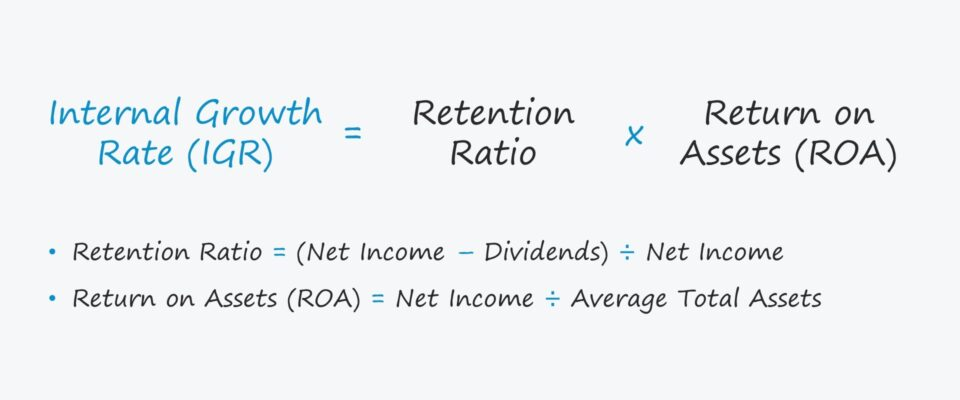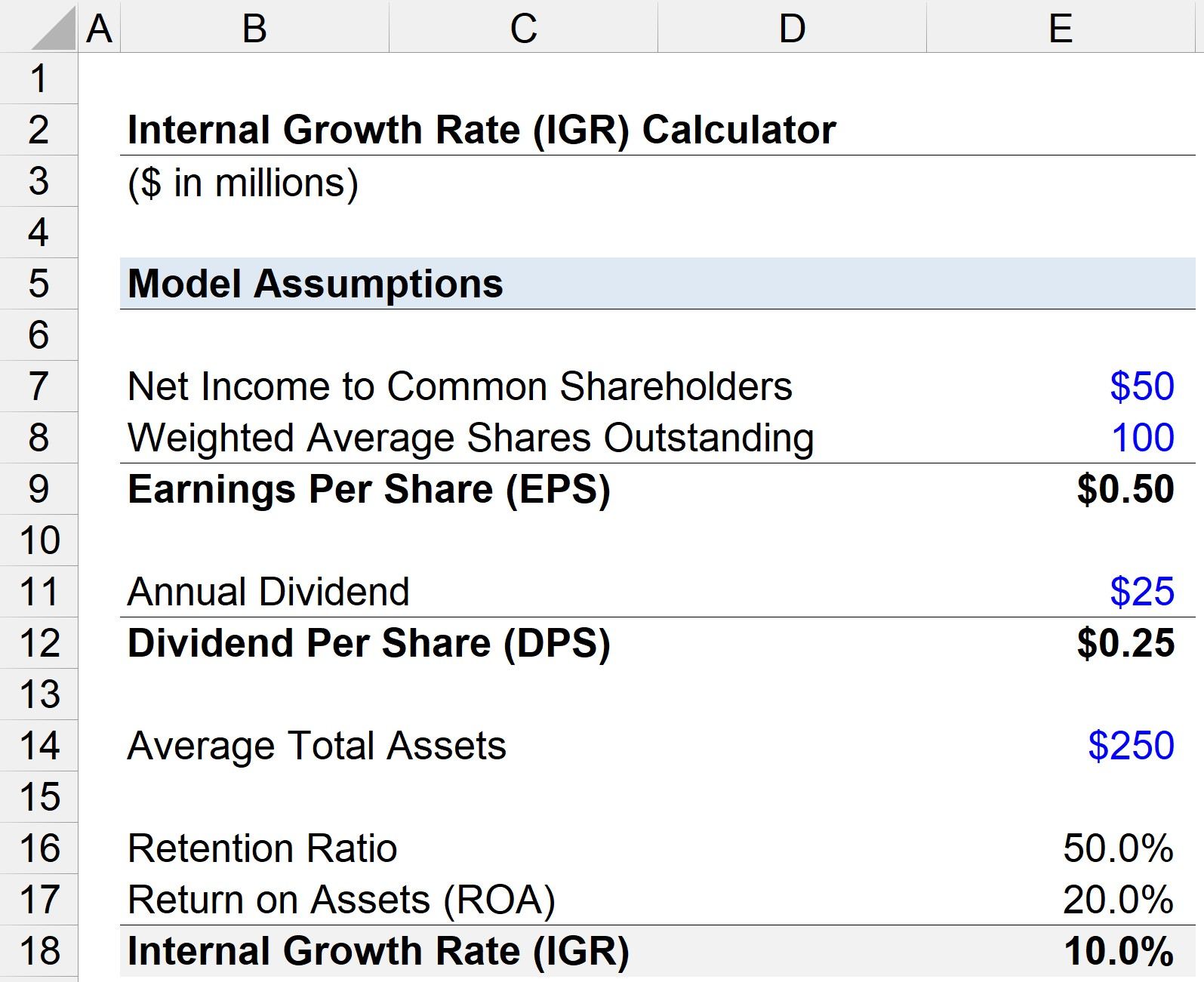# Internal Growth Rate (IGR)

Understand the Internal Growth Rate (IGR) Concept• What is the definition of the internal growth rate (IGR)?
• What does the internal growth rate (IGR) measure?
• Which formula calculates the internal growth rate (IGR)?
• How is the internal growth rate (IGR) different from the sustainable growth rate?

## Internal Growth Rate (IGR) Formula

The internal growth rate is the highest growth rate that can be achieved by a company with reliance on equity or debt issuances.

The internal growth rate (IGR) sets a “ceiling” on the maximum growth rate achievable for a specific company, assuming it does not obtain any external financing.

Instead, the implied growth rate assumes that operations are funded solely by internal sources, i.e. retained earnings.

There are two main sources of raising external financing:

1. Equity Issuances: Selling ownership stakes in the company in exchange for capital.
2. Debt Issuances: Borrowing capital with the obligation meet scheduled payments as stated in the loan agreement (e.g. interest expense, mandatory repayment at maturity)

Nowadays, practically all companies eventually must raise capital in the form of either issuing equity or debt capital (e.g. corporate bonds).

From a different viewpoint, the internal growth rate could signal that the company might need to seek external financing, i.e. more outside funding is required to reach the next growth stage.

The IGR could be sufficient for certain companies (and their investor base) while falling short of expectations for others.

## Internal Growth Rate (IGR) Formula

The formula for calculating the internal growth rate (IGR) consists of three steps:

1. Calculate the retention ratio by subtracting the annual dividend from net income and dividing that by net income
2. Calculate the return on assets (ROA) metric, which is equal to net income divided by the average total assets balance (i.e. the sum of the beginning and end of period balances divided by two)
3. Multiply the company’s retention ratio and return on assets (ROA) to arrive at the internal growth rate (IGR)
###### Internal Growth Rate Formula
• Internal Growth Rate (IGR) = Retention Ratio × Return on Assets (ROA)

Where:

• Retention Ratio = (Net Income – Dividends) ÷ Net Income
• Return on Assets (ROA) = Net Income ÷ Average Total Assets

The retention ratio is the percentage of net income that a company kept to reinvest into its operations, i.e. rather than issue a dividend to shareholders, the leftover earnings are measured by the retention ratio.

The retention ratio can also be calculated by one minus the dividend payout ratio.

• Retention Ratio = 1 – Dividend Payout Ratio

To break down the components of the internal growth rate formula in more detail, the IGR expresses the retained earnings as a percentage of total assets.

The right side of the formula can be re-arranged as:

• IGR = (Retained Earnings ÷ Net Income) × (Net Income ÷ Total Assets)
• IGR = Retention Ratio × ROA

For instance, if we assume that a company has retained earnings of \$4 million, average total assets of \$20 million, and net income of \$5 million.

• IGR = \$4 million ÷ \$20 million = 20%

After entering the same numbers into our expanded formula, the IGR is again equal to 20%.

• IGR = (\$4 million ÷ \$5 million) × (\$5 million ÷ \$20 million)
• IGR = 80% × 25% = 20%
###### Internal Growth Rate (IGR) vs Sustainable Growth Rate

One concept closely related to the internal growth rate (IGR) is the sustainable growth rate, which is the growth rate that a company could achieve if its current capital structure – i.e. the mixture of debt and equity – is maintained.

Unlike the IGR, the sustainable growth rate accounts for external financing. But the external funding sources are constrained to its existing capital structure.

In comparison, the sustainable growth rate should be higher than the internal growth rate, as more capital is available for reinvesting and discretionary spending on future growth.

## Internal Growth Rate Calculator – Excel Template

We’ll now move to a modeling exercise, which you can access by filling out the form below.Submitting ...

## Internal Growth Rate Example Calculation

Suppose a company has the following financials.

• Net Income to Common Shareholders = \$50 million
• Weighted Average Shares Outstanding = 100 million
• Annual Dividend = \$25 million

Given those assumptions, we can calculate the earnings per share (EPS) and dividend per share (DPS).

If we assume the average total assets is \$25 million, the retention ratio can be calculated by the following formula:

• Retention Ratio = (\$50 million – \$25 million) ÷ \$50 million
• Retention Ratio = 50%

Alternatively, we could divide DPS by EPS and then subtract that from one – which results in the same value, 50%.

• Retention Ratio = 1 – (DPS ÷ EPS)
• Retention Ratio = 1 – (\$0.25 ÷ \$0.50) = 50%

The final input left is the return on assets (ROA), which we calculate by dividing the net income by average total assets.

• Return on Assets (ROA) = \$50 million ÷ \$250 million
• ROA = 20%

We can now multiply the retention ratio by ROA to calculate the internal growth rate (IGR).

• Internal Growth Rate (IGR) = 50% × 20%
• IGR = 10%

The 10% IGR in our illustrative scenario implies that our company can achieve a maximum 10% growth rate without any reliance on external financing.Step-by-Step Online Course

#### Everything You Need To Master Financial Modeling

Enroll in The Premium Package: Learn Financial Statement Modeling, DCF, M&A, LBO and Comps. The same training program used at top investment banks.Inline FeedbacksLearn Financial Modeling Online

Everything you need to master financial and valuation modeling: 3-Statement Modeling, DCF, Comps, M&A and LBO.

X

The Wall Street Prep Quicklesson Series

7 Free Financial Modeling Lessons

Get instant access to video lessons taught by experienced investment bankers. Learn financial statement modeling, DCF, M&A, LBO, Comps and Excel shortcuts.